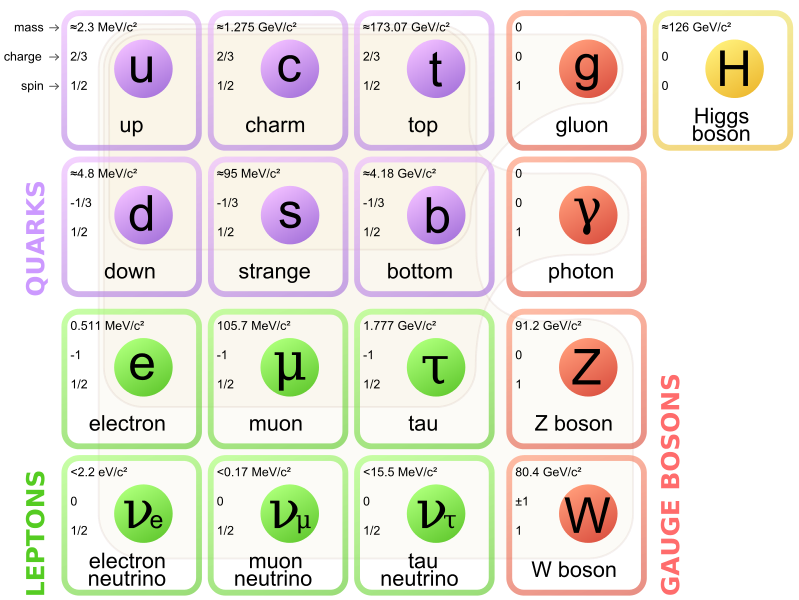Many popular science books and articles mention that the Standard Model of particle physics, the model that unifies three of the fundamental forces and describes all matter in the form of quarks and leptons, has about 18 free parameters that are not predicted by the theory.

Very few popular accounts actually tell you what these parameters are.

So here they are, in no particular order:

1. The so-called fine structure constant, $$\alpha$$, which (depending on your point of view) defines either the coupling strength of electromagnetism or the magnitude of the electron charge;
2. The Weinberg angle or weak mixing angle $$\theta_W$$ that determines the relationship between the coupling constant of electromagnetism and that of the weak interaction;
3. The coupling constant $$g_3$$ of the strong interaction;
4. The electroweak symmetry breaking energy scale (or the Higgs potential vacuum expectation value, v.e.v.) $$v$$;
5. The Higgs potential coupling constant $$\lambda$$ or alternatively, the Higgs mass $$m_H$$;
6. The three mixing angles $$\theta_{12}$$, $$\theta_{23}$$ and $$\theta_{13}$$ and the CP-violating phase $$\delta_{13}$$ of the Cabibbo-Kobayashi-Maskawa (CKM) matrix, which determines how quarks of various flavor can mix when they interact;
7. Nine Yukawa coupling constants that determine the masses of the nine charged fermions (six quarks, three charged leptons).

OK, so that’s the famous 18 parameters so far. It is interesting to note that 15 out of the 18 (the 9 Yukawa fermion mass terms, the Higgs mass, the Higgs potential v.e.v., and the four CKM values) are related to the Higgs boson. In other words, most of our ignorance in the Standard Model is related to the Higgs.

Beyond the 18 parameters, however, there are a few more. First, $$\Theta_3$$, which would characterize the CP symmetry violation of the strong interaction. Experimentally, $$\Theta_3$$ is determined to be very small, its value consistent with zero. But why is $$\Theta_3$$ so small? One possible explanation involves a new hypothetical particle, the axion, which in turn would introduce a new parameter, the mass scale $$f_a$$ into the theory.

Finally, the canonical form of the Standard Model includes massless neutrinos. We know that neutrinos must have mass, and also that they oscillate (turn into one another), which means that their mass eigenstates do not coincide with their eigenstates with respect to the weak interaction. Thus, another mixing matrix must be involved, which is called the Pontecorvo-Maki-Nakagawa-Sakata (PMNS) matrix. So we end up with three neutrino masses $$m_1$$, $$m_2$$ and $$m_3$$, and the three angles $$\theta_{12}$$, $$\theta_{23}$$ and $$\theta_{13}$$ (not to be confused with the CKM angles above) plus the CP-violating phase $$\delta_{\rm CP}$$ of the PMNS matrix.

So this is potentially as many as 26 parameters in the Standard Model that need to be determined by experiment. This is quite a long way away from the “holy grail” of theoretical physics, a theory that combines all four interactions, all the particle content, and which preferably has no free parameters whatsoever. Nonetheless the theory, and the level of our understanding of Nature’s fundamental building blocks that it represents, is a remarkable intellectual achievement of our era.

### One Response to “The parameters of the Standard Model”

1.Here I note that the fine structure constant can be derived from the structural constant of the atom, 2s0^2, s0=8.278692517. Structural constant s0, in turn, derive from the Maxwell equations: http://dx.doi.org/10.4236/jmp.2013.47121